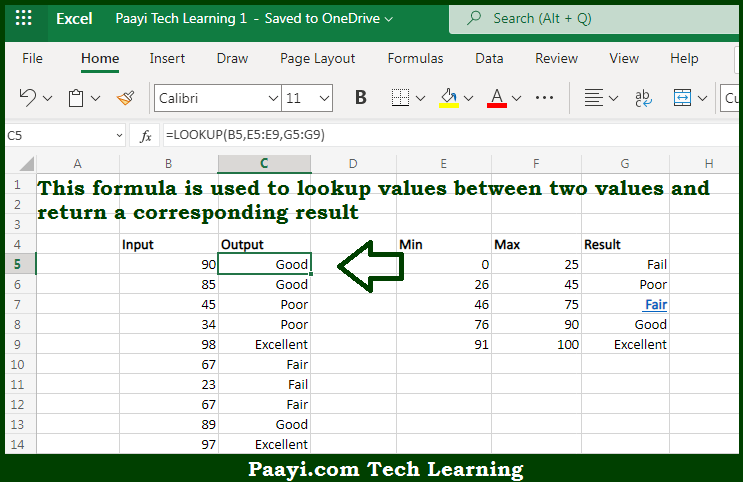# Learn How to Lookup Value Between Two Numbers in Microsoft Excel

Written by | 0 Comments | 248 Views

In this article, you will learn how to evaluate things based on the LOOKUP function in Microsoft Excel using a single/combination(s) of functions. You will also know how to Lookup Value Between Two Numbers and see the generic formula.

Learn How to Lookup Value Between Two Numbers in Microsoft Excel

The main purpose of this formula is to lookup values between two values and return a corresponding result. Here we will learn how to perform a lookup for the value between two numbers in the given data range in the workbook in Microsoft Excel. That implies, with the help of a formula based on the LOOKUP function you can able to lookup values between two values and return a corresponding result. So, with the help of this formula, you can able to perform a lookup for the value between two numbers in the given data range in the workbook in Microsoft Excel.

General Formula to Lookup Value Between Two Numbers

=LOOKUP(B5,minimums, results)

The Explanation to Lookup Value Between Two NumbersSo we know that with the help of the given formula above you can able to lookup values between two values and return a corresponding result. Here we will learn how to perform a lookup for the value between two numbers in the given data range in the workbook in Microsoft Excel. As we know the LOOKUP function is used to find the appropriate match in one range and returns the corresponding value in another.

However, in this example both maximum and minimum values are included, we only need to use the minimum values. Because if the LOOKUP function can't find a match, it will match the next smallest value. So now you have learned how to perform the lookup for the value between two numbers in the given data range in the workbook in Microsoft Excel.